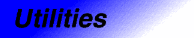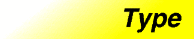# pairCategory: utilities Component type: type

## Description

`Pair<T1,T2>` is a heterogeneous pair: it holds one object of type `T1` and one of type `T2`. A pair is much like a Container, in that it "owns" its elements. It is not actually a model of Container, though, because it does not support the standard methods (such as Iterators) for accessing the elements of a Container.

Functions that need to return two values often return a `pair`.

## Example

```pair<bool, double> result = do_a_calculation();
if (result.first)
do_something_more(result.second);
else
report_error();
```

## Definition

Defined in the standard header utility, and in the nonstandard backward-compatibility header pair.h.

## Template parameters

Parameter Description Default
`T1` The type of the first element stored in the `pair`
`T2` The type of the second element stored in the `pair`

## Type requirements

`T1` and `T2` must both be models of Assignable. Additional operations have additional requirements. `Pair`'s default constructor may only be used if both `T1` and `T2` are DefaultConstructible, `operator==` may only be used if both `T1` and `T2` are EqualityComparable, and `operator<` may only be used if both `T1` and `T2` are LessThanComparable.

None.

## Members

Member Where defined Description
`first_type` `pair` See below.
`second_type` `pair` See below.
`pair()` `pair` The default constructor. See below.
`pair(const first_type&, const second_type&)` `pair` The pair constructor. See below.
`pair(const pair&)` Assignable The copy constructor
`pair& operator=(const pair&)` Assignable The assignment operator
`first` `pair` See below.
`second` `pair` See below.
`bool operator==(const pair&, const pair&)` `pair` See below.
`bool operator<(const pair&, const pair&)` `pair` See below.
```template <class T1, class T2>
pair<T1, T2> make_pair(const T1&, const T2&)
```
`pair` See below.

## New members

These members are not defined in the Assignable requirements, but are specific to `pair`.

Member Description
`first_type` The type of the pair's first component. This is a `typedef` for the template parameter `T1`
`second_type` The type of the pair's second component. This is a `typedef` for the template parameter `T2`
`pair()` The default constructor. It uses constructs objects of types `T1` and `T2` using their default constructors. This constructor may only be used if both `T1` and `T2` are DefaultConstructible.
`pair(const first_type& x, const second_type& y)` The pair constructor. Constructs a pair such that `first` is constructed from `x` and `second` is constructed from `y`.
`first` Public member variable of type `first_type`: the first object stored in the `pair`.
`second` Public member variable of type `second_type`: The second object stored in the `pair`.
```template <class T1, class T2>
bool operator==(const pair<T1,T2>& x,
const pair<T1,T2>& y);
```
The equality operator. The return value is `true` if and only the first elements of `x` and `y` are equal, and the second elements of `x` and `y` are equal. This operator may only be used if both `T1` and `T2` are EqualityComparable. This is a global function, not a member function.
```template <class T1, class T2>
bool operator<(const pair<T1,T2>& x,
const pair<T1,T2>& y);
```
The comparison operator. It uses lexicographic comparison: the return value is `true` if the first element of `x` is less than the first element of `y`, and `false` if the first element of `y` is less than the first element of `x`. If neither of these is the case, then `operator<` returns the result of comparing the second elements of `x` and `y`. This operator may only be used if both `T1` and `T2` are LessThanComparable. This is a global function, not a member function.
```template <class T1, class T2>
pair<T1, T2> make_pair(const T1& x, const T2& x)
```
Equivalent to `pair<T1, T2>(x, y)`. This is a global function, not a member function. It exists only for the sake of convenience.

## Notes

Copyright © 2006-2007 Adobe Systems Incorporated.

Use of this website signifies your agreement to the Terms of Use and Online Privacy Policy.

Search powered by Google